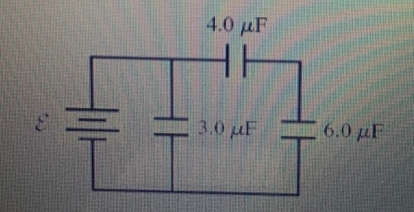# Problem: Consider the circuit shown in (Figure 1). Assume ε = 13 V. a) What is the equivalent capacitance?Express your answer with the appropriate units.b) What is the charge on 3.0μF capacitor?Express your answer with the appropriate units.c) What is the charge on 4.0μF capacitor?Express your answer with the appropriate units.d) What is the charge on 6.0μF capacitor?Express your answer with the appropriate units.

###### FREE Expert Solution

In this problem, we'll combine capacitors in series and in parallel and determine the charge stored in a capacitor.

For capacitors in series, the equivalent capacitance is:

$\overline{)\frac{\mathbf{1}}{{\mathbf{C}}_{\mathbf{e}\mathbf{q}}}{\mathbf{=}}\frac{\mathbf{1}}{{\mathbf{C}}_{\mathbf{1}}}{\mathbf{+}}\frac{\mathbf{1}}{{\mathbf{C}}_{\mathbf{2}}}{\mathbf{+}}{\mathbf{.}}{\mathbf{.}}{\mathbf{.}}{\mathbf{+}}\frac{\mathbf{1}}{{\mathbf{C}}_{\mathbf{n}}}}$

or

For two capacitors:

$\overline{){{\mathbf{C}}}_{\mathbf{e}\mathbf{q}}{\mathbf{=}}\frac{{\mathbf{C}}_{\mathbf{1}}{\mathbf{C}}_{\mathbf{2}}}{{\mathbf{C}}_{\mathbf{1}}\mathbf{+}{\mathbf{C}}_{\mathbf{2}}}}$

For capacitors in parallel, the equivalent capacitance is:

$\overline{){{\mathbf{C}}}_{\mathbf{e}\mathbf{q}}{\mathbf{=}}{{\mathbf{C}}}_{{\mathbf{1}}}{\mathbf{+}}{{\mathbf{C}}}_{{\mathbf{2}}}{\mathbf{+}}{\mathbf{.}}{\mathbf{.}}{\mathbf{.}}{\mathbf{+}}{{\mathbf{C}}}_{{\mathbf{n}}}}$

The charge stored by a capacitor:

$\overline{){\mathbf{Q}}{\mathbf{=}}{\mathbf{C}}{\mathbf{V}}}$

80% (11 ratings)###### Problem Details

Consider the circuit shown in (Figure 1). Assume ε = 13 V.

a) What is the equivalent capacitance?

Express your answer with the appropriate units.

b) What is the charge on 3.0μF capacitor?

Express your answer with the appropriate units.

c) What is the charge on 4.0μF capacitor?

Express your answer with the appropriate units.

d) What is the charge on 6.0μF capacitor?

Express your answer with the appropriate units.Frequently Asked Questions

What scientific concept do you need to know in order to solve this problem?

Our tutors have indicated that to solve this problem you will need to apply the Combining Capacitors in Series & Parallel concept. You can view video lessons to learn Combining Capacitors in Series & Parallel. Or if you need more Combining Capacitors in Series & Parallel practice, you can also practice Combining Capacitors in Series & Parallel practice problems.